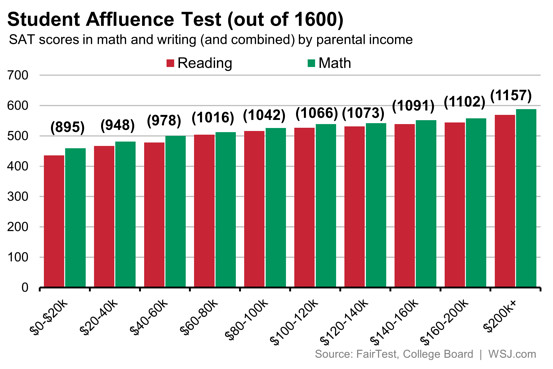Earlier, I talked about this chart showing SAT scores of kids from different household income levels:In this post, I will estimate the IQ of the adults of various income levels from how their kids score on the SAT. Now the first step is converting the combined SAT scores of the kids of all ten income levels into IQ using this formula I created:

IQ = 39.545 + 0.068(SAT score)

This gives the following IQ’s for the ten income groups: 100, 104, 106, 109, 110, 112, 113, 114, 114, 118

One problem is mostly the brightest kids tend to take the SAT. For example, the average SAT taker has combined score (reading + math) of 1010, equivalent to an IQ of 108 (8 points higher than the national average). If we very crudely assume that at every income level, the ones who take the SAT are 8 points above the norms for their income group, we should correct these IQ’s by subtracting 8 points. Thus the IQ’s for the ten income groups become: 92, 96, 98, 101, 102, 104, 105, 106, 106, 110

Of course I’m not interested in the IQs of the kids from different income groups. I’m interested in the IQs of their parents since they’re the ones who earned the income. In order to estimate the IQ’s of the parents from the IQs of the kids, we note that the IQ correlation between both parents and their kid is 0.6 (by late adolescence/adulthood). This means that the children of exceptional parents will be only 60% as exceptional as their parents, so to estimate the IQs of the parents, we simply divide the number of IQ points the kids score above or below 100 by 0.6 to determine how far above and below average their parents are. This gives the following IQ’s for the parents in the ten income groups: 87, 93, 97, 102, 103, 107, 108, 110, 110, 117

The following chart shows the IQs of the parents of all ten income groups along with the income percentile of each income group calculated here:It is interesting to ask how linear the correlation between IQ and income is. In an absolute sense it’s not linear at all because IQ is normally distributed and income has an extremely skewed distribution, especially at the high end. But what happens when we convert income into the same scale as IQ (a normal distribution with a mean of 100 and an SD of 15)? This is easily done by taking various income percentiles and assigning them MQs (Money Quotients) based on their position in a normalized distribution with a mean of a 100 and and SD of 15. So the median household earning over \$200,000 a year is at the 97 percentile and thus is assigned an MQ of 128. Households earning around \$0-20K a year are at the 11 percentile, thus assigned an MQ of 82. Thus we can graph the the average IQ of earners from various MQs and note how linear the graph is: